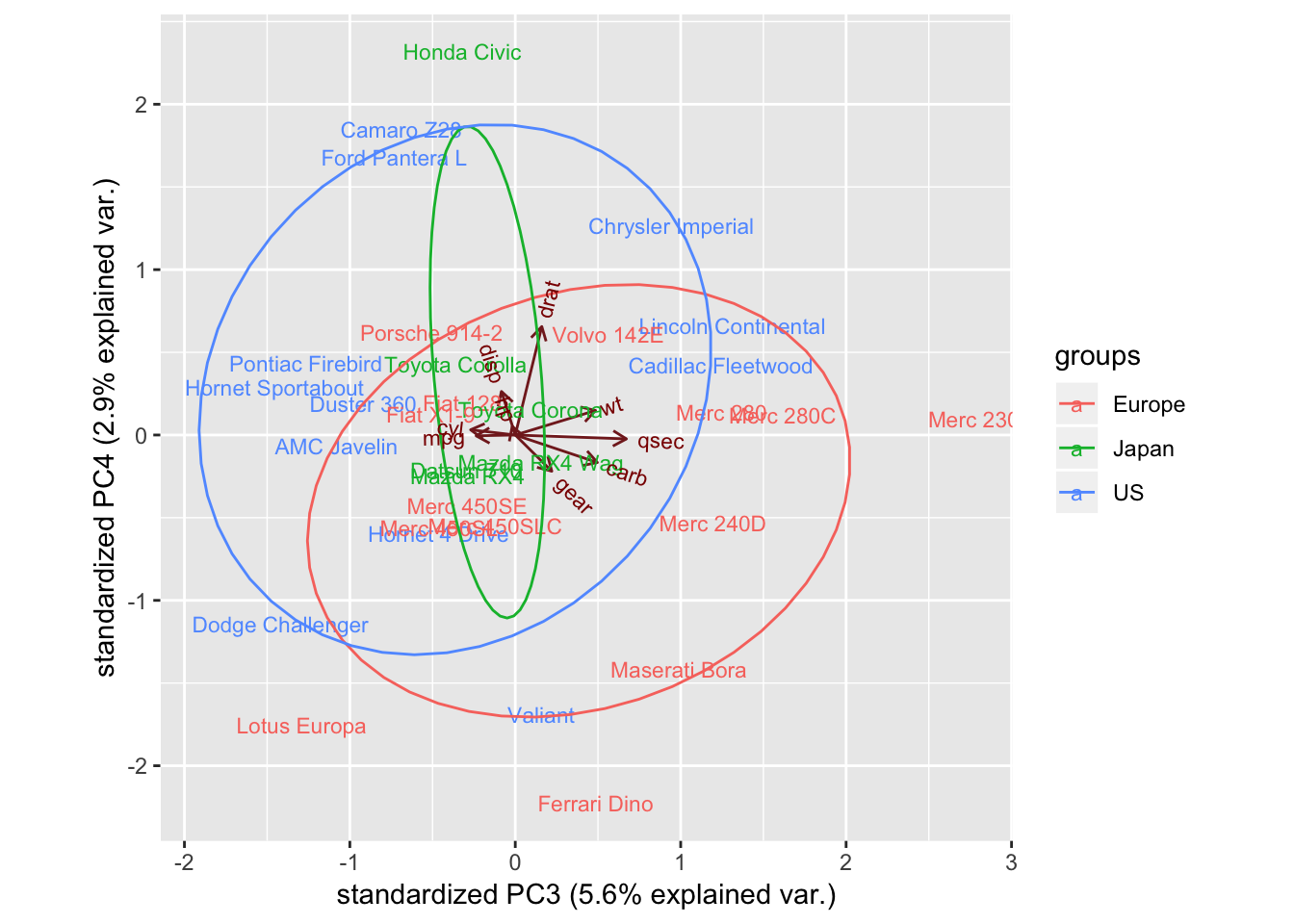# 主成分分析

### 2018/12/18

#### PCA原理 - 奇异值分解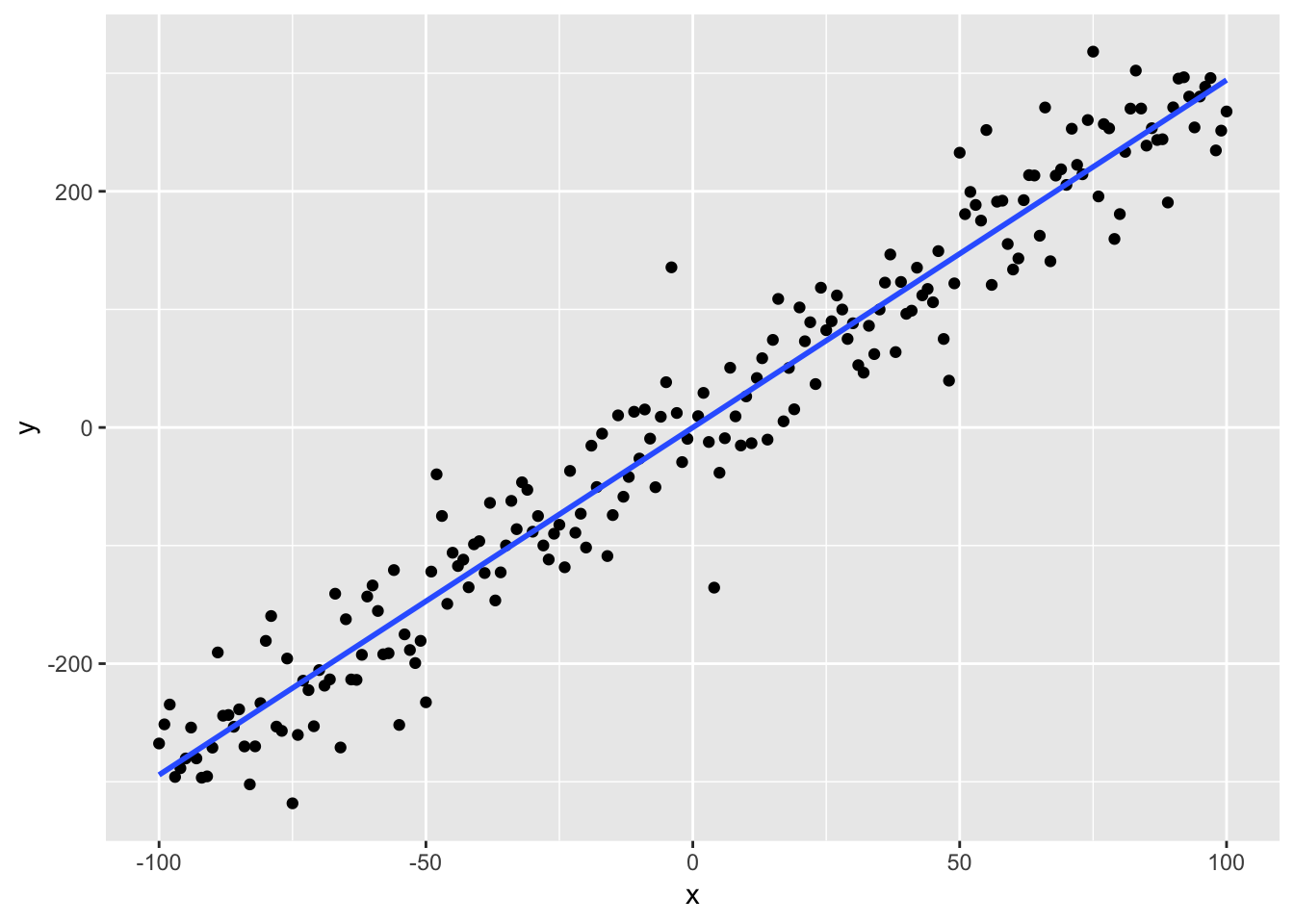$$AA^T$$构成一个$$2 \times 2$$的矩阵，其中$$(AA^T)_{11}$$$$(AA^T)_{22}$$是行向量的方差，而$$(AA^T)_{12}$$$$(AA^T)_{21}$$即是两行的协方差。因此，协方差矩阵可表示为

$S = \frac {AA^T} {n-1}$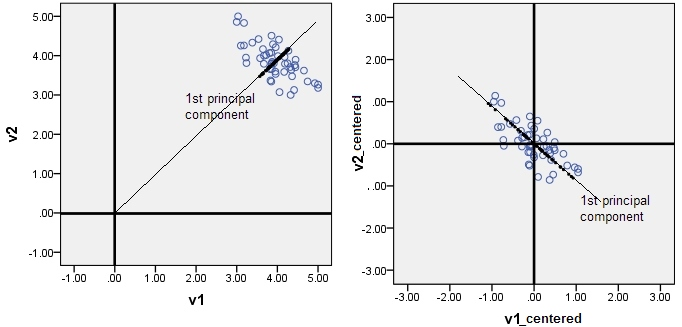$A = \sqrt{\sigma_1}u_1v_1^T + \sqrt{\sigma_2}u_2v_2^T$

$A = \left[ \begin{matrix} 3 & -4 & 7 & 1 & -4 & -3 \\ 7 & -6 & 8 & -1 & -1 & -7 \end{matrix} \right] \qquad S = \frac{AA^T}{5} = \left[ \begin{matrix} 20 & 25 \\ 25 & 40 \end{matrix} \right]$

$$S$$特征值为57和3，那么$$A$$可表示为

$A = \sqrt{57}u_1v_1^T + \sqrt{3}u_2v_2^T$

$$u_1$$对应于散点图变化最大的方向（直线），$$u_2$$垂直于$$u_1$$，表征垂直于直线方向上的小幅摆动。

### PCA数学推导

$\bar{x} = \frac{\sum_{n=1}^{N}}{N}$ 原始数据向投影向量$$u$$投影之后的方差为：

$\frac{1}{N} \sum_{n-1}^{N}(u^Tx_n - u^T\bar{x})^2 = u^TSu$ 根据梯度优化原则（拉格朗日乘子）:

$u^TSu + \lambda(1-u^Tu) = 0$ $Su = \lambda u$

#### 相关系数矩阵

$Cov(X, Y) = E((X - E(X))(Y - E(Y)))$

$Cor(X, Y) = \frac {Cov(X,Y)}{\sqrt{D(X)}\sqrt{D(Y)}}$

$X^* = \frac{X - E(X)}{\sqrt{D(x)}} Y^* = \frac{Y - E(Y)}{\sqrt{D(Y)}}$

$Cov(X^*, Y^*) = \frac {E((X - E(X))(Y - E(Y)))}{\sqrt{D(X)}\sqrt{D(Y)}} = \frac {Cov(X,Y)}{\sqrt{D(X)}\sqrt{D(Y)}} = Cor(X, Y)$

#### 主成分分析R代码实现

mtcars数据为例，PCA是针对数值数据，所以我们删除mtcars中的分类变量vsam

library(dplyr)
# 首先中心化，因为各变量量纲不同scale = TRUE,
# 主成分是原始变量的线性组合，\$rotation表征了线性组合系数，x
# 表示新的坐标值
mtcars_pca <- prcomp(select(mtcars, -c("vs", "am")),
center = TRUE, scale = TRUE)

summary(mtcars_pca)
## Importance of components:
##                           PC1    PC2     PC3     PC4     PC5     PC6
## Standard deviation     2.3782 1.4429 0.71008 0.51481 0.42797 0.35184
## Proportion of Variance 0.6284 0.2313 0.05602 0.02945 0.02035 0.01375
## Cumulative Proportion  0.6284 0.8598 0.91581 0.94525 0.96560 0.97936
##                            PC7    PC8     PC9
## Standard deviation     0.32413 0.2419 0.14896
## Proportion of Variance 0.01167 0.0065 0.00247
## Cumulative Proportion  0.99103 0.9975 1.00000

##### PCA结果可视化

biplot显示样本在新的坐标下的位置，同时显示原始的变量值(根据rotation在相应的主成分标出原始值)，向量箭头起始于中心点。

library(ggbiplot)
ggbiplot(mtcars_pca, labels=rownames(mtcars))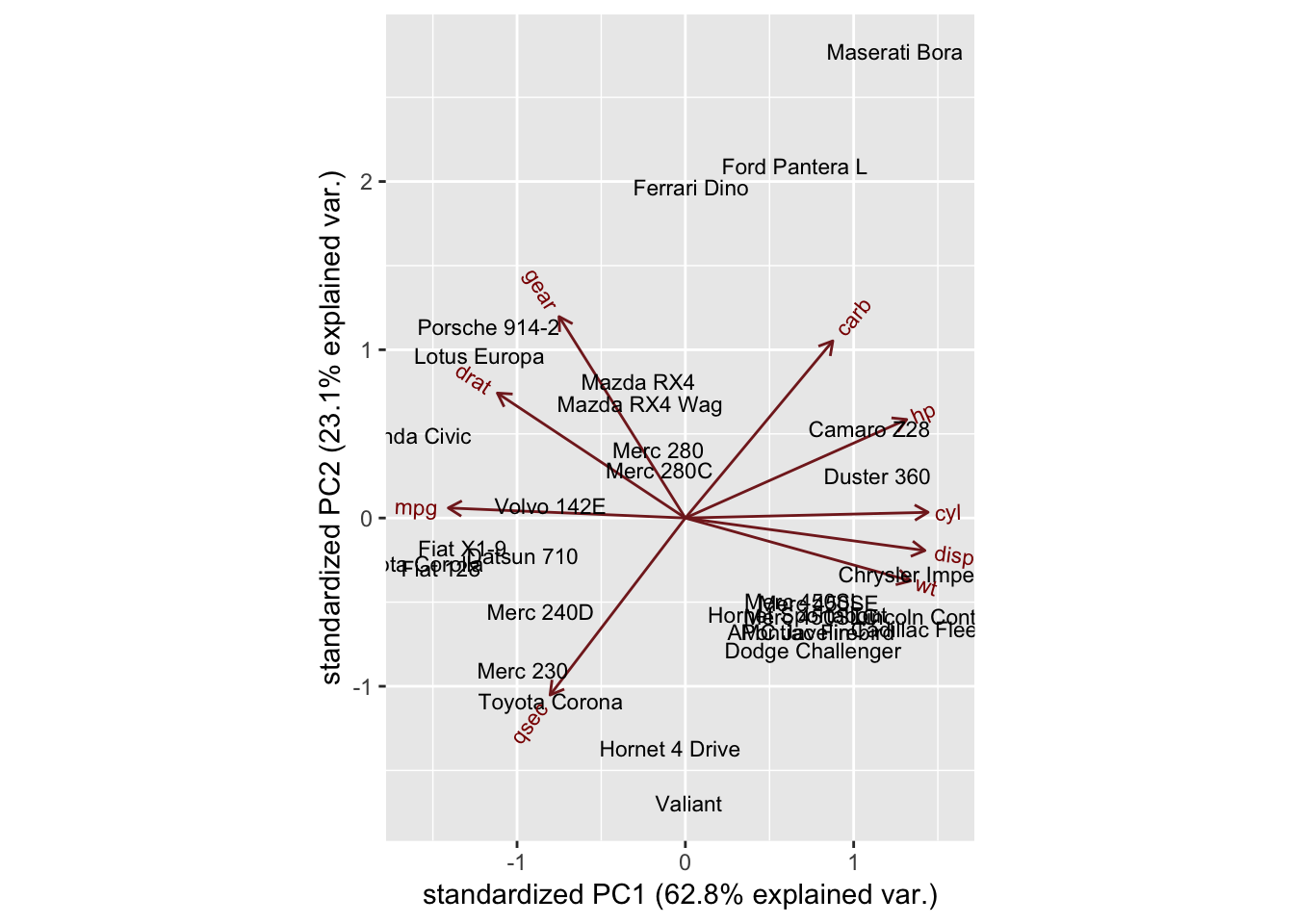##### 结果解读

mtcars_country <- c(rep("Japan", 3), rep("US",4), rep("Europe", 7),rep("US",3), "Europe", rep("Japan", 3), rep("US",4), rep("Europe", 3), "US", rep("Europe", 3))

ggbiplot(mtcars_pca,ellipse=TRUE,  labels=rownames(mtcars), groups=mtcars_country)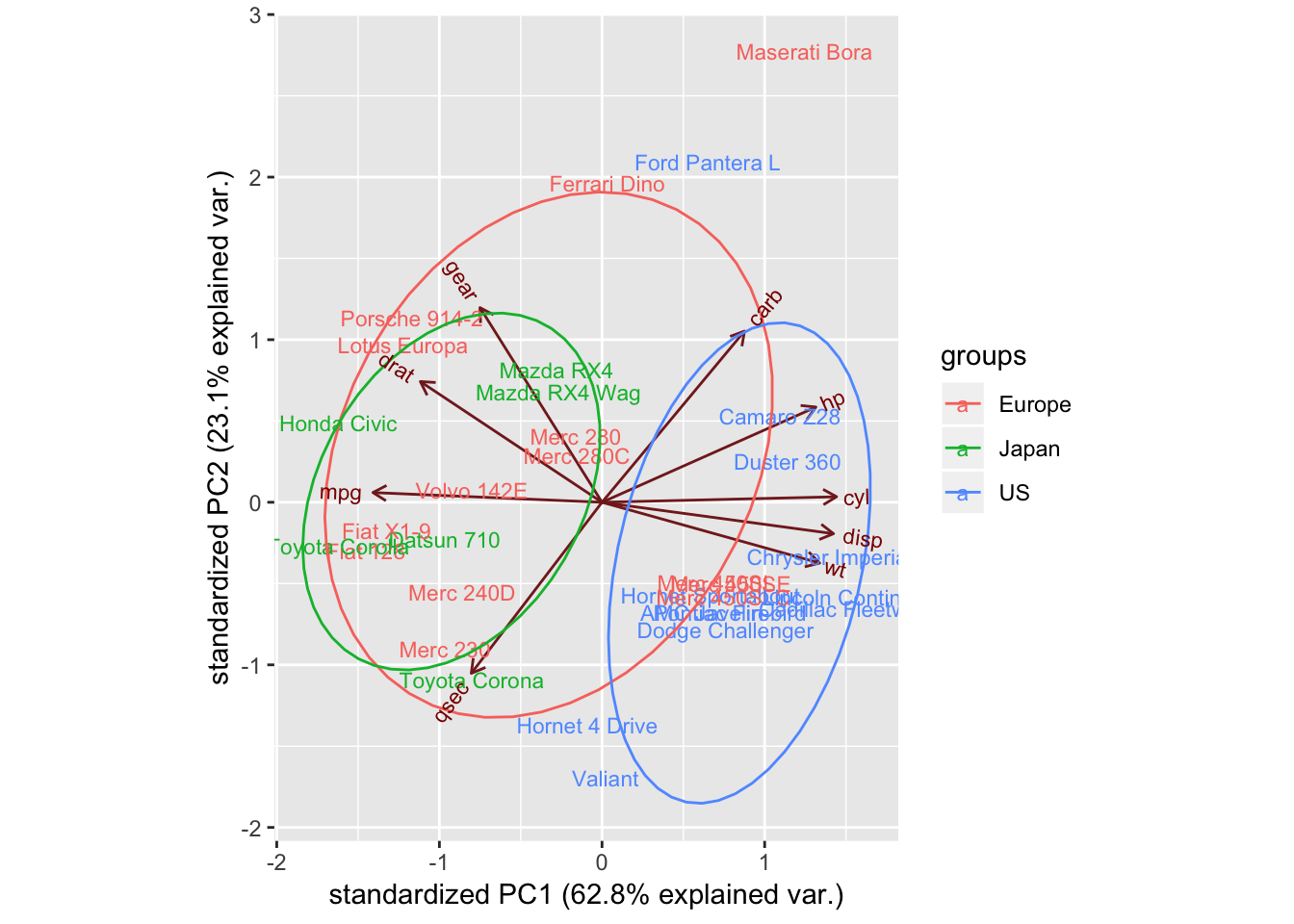ggbiplot(mtcars_pca,ellipse=TRUE,choices=c(3,4),   labels=rownames(mtcars), groups=mtcars_country)